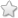# TNSB - 8 - Maths :: Algebra - Term 2

## Course Content / Subtopic list:

1. "Introduction - Forming Algebraic Expressions - Translate A Few Statements Into An Algebraic Language And Recall How To Frame Expressions, Linear Equation Used To Find Unit Price And Total, Linear Equation Used For Speed, Distance, Time And Average Speed"
2. Equations - Linear Equations In One Or More Variable, Solutions Of A Linear Equation
3. Word Problem That Involve Linear Equations - Examples
4. Graph - Introduction, Graph Sheets, Signs In The Graphs, Quadrants, Plotting The Given Points On Graph
5. Linear Graph - Linear Pattern, Graph Of A Linear Function In Two Variables, Applications
0.00(0 votes)